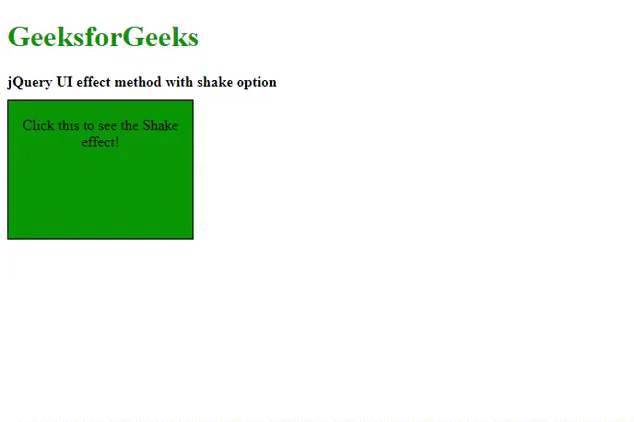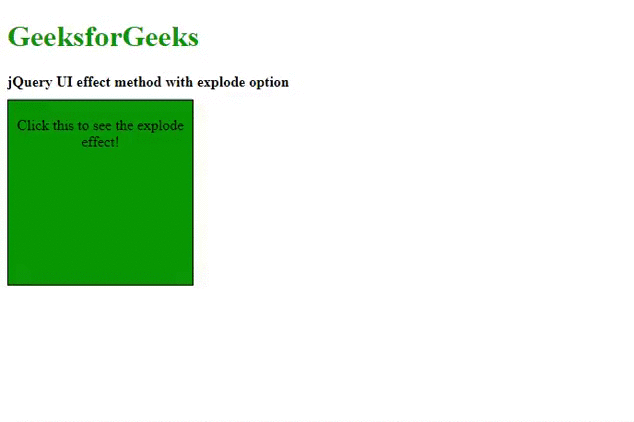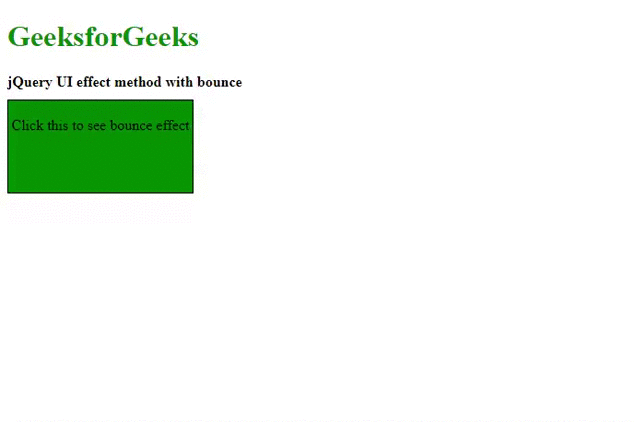# jQuery UI | effect() Method

jQuery UI framework provides effect() method for managing different variety of visual effects on the selected elements without using show() and hide() methods. There are many types of effects which are passed as a parameter to the effect() method.

Syntax:

`\$(selector).effect(effectType[, options ] [, duration ] [, complete ]);`

Parameters: This method accepts four parameters as mentioned above and described below:

• effectType: The type of effect which is chosen for the visual transition of the selected element.
• options: The options or settings are used for easing effects.
• duration: The time or duration in milliseonds used for the animation effect to run. The default value is 400ms.
• complete: This is the callback function which is executed when the visual effect is completed.

href=”https://code.jquery.com/ui/1.10.4/themes/ui-lightness/jquery-ui.css”rel=”stylesheet”type=”text/css”/>
<script src=”https://code.jquery.com/jquery-1.10.2.js”></script>
<script src=”https://code.jquery.com/ui/1.10.4/jquery-ui.js”></script>

There are many variety of effectType which can be passed to the effect() method as per the project requirements. In the following example, we will be only demonstrating some of them along with output images.

Example 1: The following example demonstrates jQuery UI effect() method with shake effect with options settings as time and distance.

 ` ` `<``html` `lang``=``"en"``> ` ` `  `<``head``> ` `    ``<``meta` `charset``=``"utf-8"``> ` `    ``<``meta` `name``=``"viewport"` `content``= ` `        ``"width=device-width, initial-scale=1"``> ` ` `  `    ``<``title``>jQuery UI effect method ` ` `  `    ``<``link` `href``= ` `"http://code.jquery.com/ui/1.10.4/themes/ui-lightness/jquery-ui.css"` `        ``rel``=``"stylesheet"``> ` ` `  `    ``<``script` `src``= ` `"http://code.jquery.com/jquery-1.10.2.js"``> ` `    `` ` ` `  `    ``<``script` `src``= ` `"http://code.jquery.com/ui/1.10.4/jquery-ui.js"``> ` `    `` ` ` `  `    ``<``style``> ` `        ``#divID { ` `            ``width: 200px; ` `            ``height: 150px; ` `            ``text-align: center; ` `            ``background: green; ` `            ``border: 1px solid black; ` `        ``} ` ` `  `        ``.height { ` `            ``height: 10px; ` `        ``} ` `    `` ` ` `  `    ``<``script``> ` `        ``\$(document).ready(function () { ` `            ``\$('#divID').click(function () { ` `                ``\$("#divID").effect("shake", { ` `                    ``times: 20, ` `                    ``distance: 180 ` `                ``}, 3000, function () { ` `                    ``\$("#divID").css( ` `                        ``"background", "#ff0000"); ` `                ``}); ` `            ``}); ` `        ``});   ` `    `` ` ` ` ` `  `<``body``> ` `    ``<``h1` `style``=``"color:green"``>GeeksforGeeks ` ` `  `    ``<``b``>jQuery UI effect method with shake option ` `     `  `    ``<``div` `class``=``"height"``> ` `     `  `    ``<``div` `id``=``"divID"``> ` `        ``<``span``> ` `            ``Click this to see the Shake effect! ` `        `` ` `    `` ` ` ` ` `  ` `

Output:Example 2: The following code demonstrates jQuery UI effect() method with explode effect with easing setting as “swing”.

 ` ` `<``html` `lang``=``"en"``> ` ` `  `<``head``> ` `    ``<``meta` `charset``=``"utf-8"``> ` `    ``<``meta` `name``=``"viewport"` `content``= ` `            ``"width=device-width, initial-scale=1"``> ` ` `  `    ``<``title``>jQuery UI effect with explode ` ` `  `    ``<``link` `href``= ` `"http://code.jquery.com/ui/1.10.4/themes/ui-lightness/jquery-ui.css"` `        ``rel``=``"stylesheet"``> ` ` `  `    ``<``script` `src``= ` `"http://code.jquery.com/jquery-1.10.2.js"``> ` `    `` ` ` `  `    ``<``script` `src``= ` `"http://code.jquery.com/ui/1.10.4/jquery-ui.js"``> ` `    `` ` ` `  `    ``<``style``> ` `        ``#divID { ` `            ``height: 200px; ` `            ``width: 200px; ` `            ``text-align: center; ` `            ``background: green; ` `            ``border: 1px solid black; ` `        ``} ` ` `  `        ``.height { ` `            ``height: 10px; ` `        ``} ` `    `` ` ` `  `    ``<``script``> ` `        ``\$(document).ready(function () { ` `            ``\$('#divID').click(function () { ` `                ``\$("#divID").effect({ ` `                    ``effect: "explode", ` `                    ``easing: "swing", ` `                    ``pieces: 12, ` `                    ``duration: 3000 ` `                ``}); ` `            ``}); ` `        ``});   ` `    `` ` ` ` ` `  `<``body``> ` `    ``<``h1` `style``=``"color:green"``>GeeksforGeeks ` ` `  `    ``<``b``>jQuery UI effect method with explode option ` `     `  `    ``<``div` `class``=``"height"``> ` `     `  `    ``<``div` `id``=``"divID"``> ` `        ``<``span``> ` `            ``Click this to see the explode effect! ` `        `` ` `    `` ` ` ` ` `  ` `

Output:Example 3: The following example demonstrates the effect() method with bounce effect type.

 ` ` `<``html` `lang``=``"en"``> ` ` `  `<``head``> ` `    ``<``meta` `charset``=``"utf-8"``> ` `    ``<``meta` `name``=``"viewport"` `content``= ` `        ``"width=device-width, initial-scale=1"``> ` `         `  `    ``<``title``>jQuery UI effect Method with bounce ` ` `  `    ``<``link` `href``= ` `"http://code.jquery.com/ui/1.10.4/themes/ui-lightness/jquery-ui.css"` `        ``rel``=``"stylesheet"``> ` ` `  `    ``<``script` `src``= ` `"http://code.jquery.com/jquery-1.10.2.js"``> ` `    `` ` ` `  `    ``<``script` `src``= ` `"http://code.jquery.com/ui/1.10.4/jquery-ui.js"``> ` `    `` ` ` `  `    ``<``style``> ` `        ``#divID { ` `            ``height: 100px; ` `            ``width: 200px; ` `            ``background: green; ` `            ``text-align: center; ` `            ``border: 1px solid black; ` `        ``} ` ` `  `        ``.height { ` `            ``height: 10px; ` `        ``} ` `    `` ` `    ``<``script``> ` `        ``\$(document).ready(function () { ` `            ``\$('#divID').click(function () { ` `                ``\$("#divID").effect("bounce", { ` `                    ``times: 20, ` `                    ``distance: 200 ` `                ``}, 3000, function () { ` `                    ``\$(this).css("background", "#ff0000"); ` `                ``}); ` `            ``}); ` `        ``});   ` `    `` ` ` ` ` `  `<``body``> ` `    ``<``h1` `style``=``"color:green"``>GeeksforGeeks ` `    ``<``b``>jQuery UI effect method with bounce ` `    ``<``div` `class``=``"height"``> ` `    ``<``div` `id``=``"divID"``> ` `        ``<``span``> ` `            ``Click this to see bounce effect ` `        `` ` `    `` ` ` ` ` `  `   `

Output:My Personal Notes arrow_drop_upCheck out this Author's contributed articles.

If you like GeeksforGeeks and would like to contribute, you can also write an article using contribute.geeksforgeeks.org or mail your article to contribute@geeksforgeeks.org. See your article appearing on the GeeksforGeeks main page and help other Geeks.

Please Improve this article if you find anything incorrect by clicking on the "Improve Article" button below.